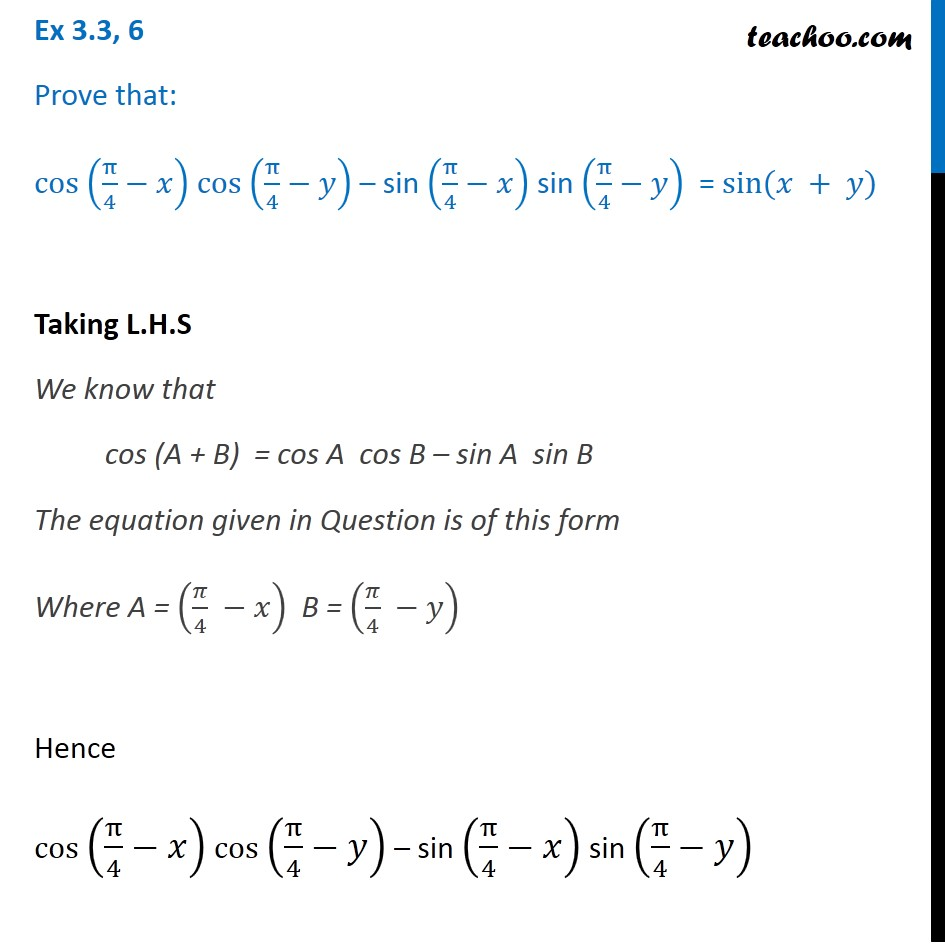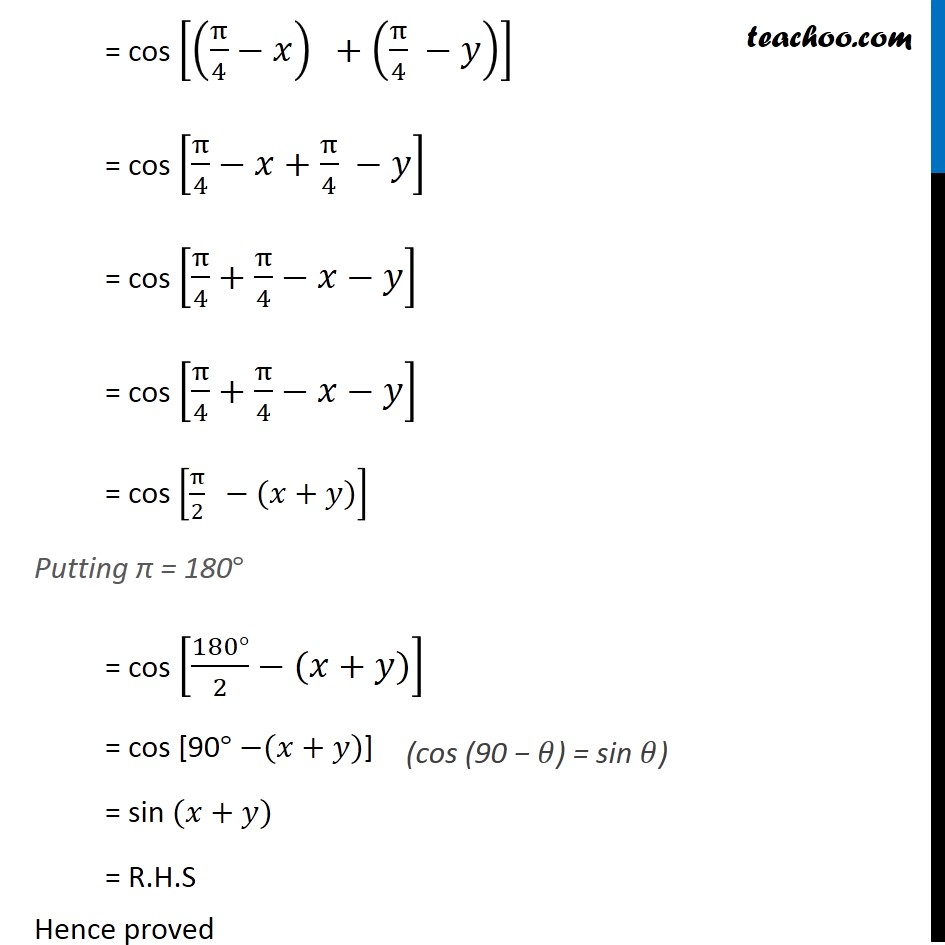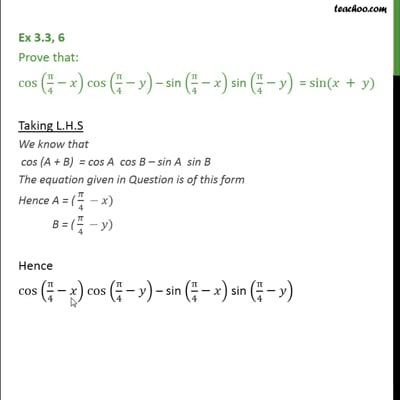(x + y) formula

Chapter 3 Class 11 Trigonometric Functions
Concept wiseThis video is only available for Teachoo black users

Introducing your new favourite teacher - Teachoo Black, at only ₹83 per month

### Transcript

Ex 3.3, 6 Prove that: cos (π/4−𝑥) cos (π/4−𝑦) – sin (π/4−𝑥) sin (π/4−𝑦) = sin⁡(𝑥 + 𝑦) Taking L.H.S We know that cos (A + B) = cos A cos B – sin A sin B The equation given in Question is of this form Where A = (𝜋/4 −𝑥) B = (𝜋/4 −𝑦) Hence cos (π/4−𝑥) cos (π/4−𝑦) – sin (π/4−𝑥) sin (π/4−𝑦) = cos [(π/4−𝑥)" " +(π/4 −𝑦)] = cos [π/4−𝑥+π/4 −𝑦] = cos [π/4+π/4−𝑥−𝑦] = cos [π/4+π/4−𝑥−𝑦] = cos [π/2 " " −(𝑥+𝑦)] Putting π = 180° = cos [(180°)/2−(𝑥+𝑦)] = cos [90° −(𝑥+𝑦)] = sin (𝑥+𝑦) = R.H.S Hence proved